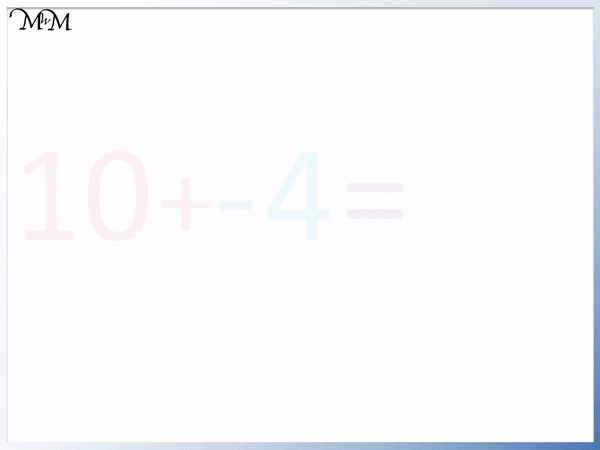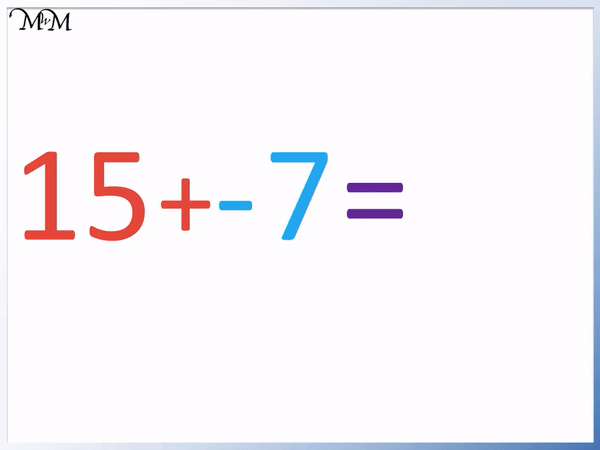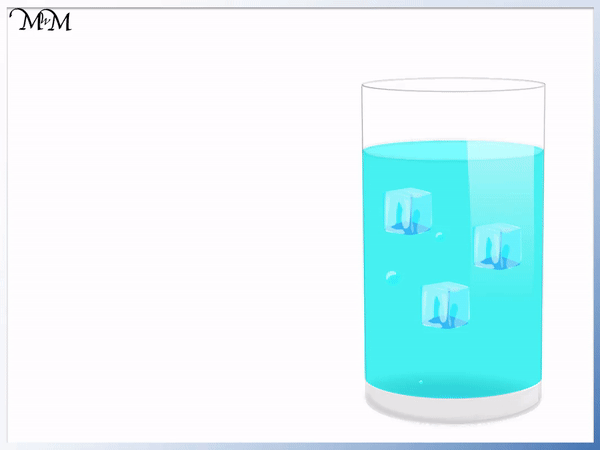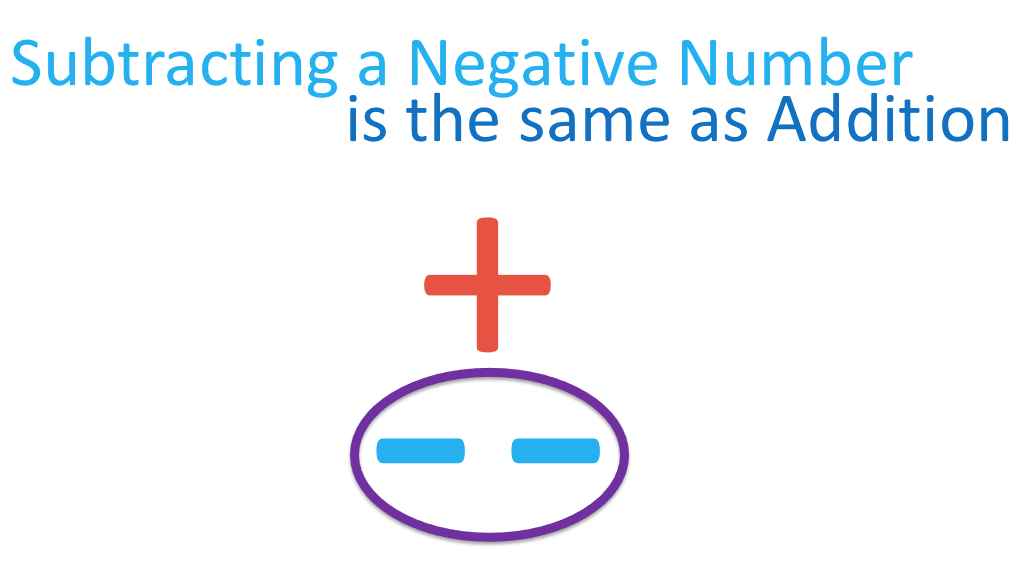# Adding and Subtracting Negative Numbers• Here is a guide for understanding the rules for adding and subtracting negative numbers.
• If two signs that are next to each other are the same, then they can be replaced by a plus sign.
• Both ‘- -‘ and ‘+ +’ are replaced with a single plus sign.
• If two signs that are next to each other are different, then they can be replaced with a minus sign.
• Both ‘+ -‘ and ‘- +’ can be replaced with a single minus sign.
Two positives or two negatives are the same as addition.

A negative and a positive in any order are the same as a subtraction.• Whenever we have a positive and negative sign next to each other we simply replaced both signs with a single subtraction.
• We replace the ‘+ -‘ or ‘- +’ with a ‘-‘.
• 10 + – 4 can be rewritten as 10 – 4.
• 10 – 4 = 6, therefore 10 + – 4 = 6.
• 17 – + 6 can be rewritten as 17 – 6.
• 17 – 6 = 11, therefore 17 – + 6 = 11.Supporting Lessons

#### Subtracting Negative Numbers# How to Add and Subtract Negative Numbers

## Rules for Adding and Subtracting Negative Numbers

The rule for adding and subtracting negative numbers is to add the numbers if the signs are the same and to subtract the numbers if the signs are different.

If there are two negative signs or two postive signs, then perform an addition. If there is a positive and negative sign in any order, then perform a subtraction.

Here is a guide to the rules for adding and subtracting directed numbers.We can think of the negative sign as having an opposite effect. A negative sign will change the sign next to it to have the opposite effect.

In the case of ‘- -‘, the first negative sign changes the negative sign it is next to into a ‘+’. There are four rules for adding and subtracting negative numbers, which are:

 Same signs Signs Rule + and + – and – + and – – and +

These rules are used when there are two signs next to each other in an equation.

For example, 10 + – 4 can be written as 10 – 4, which equals 6.

17 – + 6 can be written as 17 – 6, which equals 11.In these examples, the combination of ‘+ -‘ or ‘- +’ were replaced with a single subtraction.

Here is an example of subtracting a negative number. We have 2 – – 5. The two signs ‘- -‘ are the same, so we will be performing an addition.

Two negative signs are replaced with a single addition.2 – – 5 is the same as 2 + 5, which equals 7.

The final case shows two positive signs being replaced with one.

For example, 6 + + 3 is the same as 6 + 3, which equals 9.

There is no need to write two plus signs in this sum.

To add a negative number, simply ignore the negative sign and subtract it from the other number instead of adding it.

For example, here is 15 + – 7. We have a positive number add a negative number. We are adding negative 7 to positive 15.Replace the ‘+ -‘ in a sum with a single ‘-‘ sign.

15 + – 7 is the same as 15 – 7.

15 – 7 = 8 and so, 15 + – 7 = 8.

To understand why adding a negative number is the same as a subtraction, we can consider negative numbers as ice cubes. By adding an ice cube, the temperature drops. By adding a negative, the value of the number drops.

Here is a drink that measures 7 degrees.We will think of our negative number (-1) as an ice cube.

Adding an ice cube makes the drink colder and so, the temperature goes down.

7 – 1 = 6 and so, adding the ice cube resulted in a subtraction.

We can write 7 + – 1 = 6 or we can write the negative number in brackets as 7 + (-1) = 6.

It does not matter if a negative number is written in brackets or not. Writing the bracket can make it easier for some people to visualise the negative number in this ice cube analogy and also to show that the negative sign belongs to the 1. However, not writing the bracket can make it easier to see the ‘+ -‘ combination and more easily replace it with a ‘-‘.Each ice cube lowers the temperature by 1. Each ice cube is worth (-1).

The drink was 7 degrees to begin with. Adding three ice cubes can be shown with the sum 7 + – 3.

The temperature goes down by 3 to get to 4.

7 + – 3 = 4 because 7 – 3 = 4.

It does not matter which order the positive and negative signs are written. Both + – and – + are replaced with a -. If there is a positive and negative sign together, the result is a subtraction.

Here is an example of 8 – 5. The 5 can be written as positive 5 or + 5.

We can write 8 – 5 as 8 – + 5 or even as 8 – (+ 5). We don’t normally write the plus sign in front of the 5 because we assume a number is positive unless told otherwise.8 – 5 = 3 and so 8 – + 5 is also equal to 3.

The ‘- +’ is the same as a ‘-‘ sign. The ‘- +’ is replaced with a subtraction.

## Subtracting a Negative Number

To subtract a negative number from another number, ignore any negative signs and add the numbers together.

For example, here is 10 – – 4. Ignoring the negative signs, we have 10 and 4. We add 10 and 4 to get 14. Therefore, 10 – – 4 = 14.If two negative signs are next to each other, we can replace them with a positive sign. ‘- -‘ can be replaced with a ‘+’.

Here is another example of subtracting a negative number. We have 8 – – 3.

It can be helpful to circle any double negatives and write an addition sign above.8 – – 3 can be written as 8 + 3, which equals 11. 8 – – 3 = 11.

To understand why subtracting a negative number results in an addition, we can consider the negative number as an ice cube. Removing an ice cube can make the temperature go up. Removing a negative number can make the value of the number go up.

Here is a drink which contains an ice cube. Its temperature is 5 degrees.If the ice cube is removed, the temperature will increase. If the ice cube is cooling the drink by 1 degree, then removing it will warm up the drink by 1 degree.

The drink was 5 degrees and is now 6 degrees. 5 + 1 = 6 and so 5 – – 1 = 6.

We can see that the ‘- -‘ in 5 – – 1 = 6 can be replaced with a ‘+’ to make 5 + 1 = 6.

Here is a different drink which contains 3 ice cubes. It is currently 5 degrees.

We will remove all three ice cubes to make the temperature go up by three.5 + 3 = 8 and so, 5 – – 3 = 8.

Two negative signs make a positive because one negative sign creates the opposite effect in the other negative sign. The effects in each of the two negative signs cancel out to produce a positive effect.

## Teaching Adding and Subtracting Directed Numbers

To teach directed numbers, it is important to begin with real life analogies. Some examples include:

• Removing an ice cube causing the temperature of a drink to increase.
• Removing a weight can cause something to go upwards
• Removing a bill or debt means that you have more money to spend.

When teaching adding and subtracting negative numbers in general, it is useful to encourage the circling of the combinations of positive and negative signs before replacing them with a + or – sign. This can build up a longer term strategy which is not so easily forgotten.

With several different negative number rules to remember, it is important to get lots of practice with each method and having written strategies can help with this.Children can sometimes forget to use this procedure and could believe the sum to be more complicated than it is. By getting them in the habit of circling the two signs and replacing them with a single operation, there is more structure for tackling the question.Now try our lesson on How to Subtract Positives from Negatives Using a Number Line where we learn how to subtract a positive number from a number that is already negative.error: Content is protected !!